## REACTIONS IN EQUILIBRIUM

#### Introduction

Every chemical reaction starts with reactants (the reacting substances)in order to make products.
This seems as simple as that, but it is not always like that. In practice, most products have the tendency to react back, to reproduce the old reactants.
In such cases we have a 'forth-and-back' reaction.

Example:
the 'forth'reaction:     I2+ H2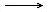2HI

the 'back'reaction:     2HI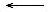I2+ H2       or:       I2 + H22HI

The habits in chemistry are to combine those two arrows, the two reactions in one reaction equarion with a double arrow:

I2+ H2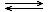2HI (ΔH < 0)
Another habit in chemistry is to add the energy effect to the right side of the equation, as it belongs to the forth-reaction:
ΔH > 0 or ΔH < 0.
In the above example, ΔH < 0 means that the forth reaction is exothermic.

The same equilibrium can also be written as follows:

2HII2+ H 2     (ΔH > 0)

Content

## 1. Reversibility and yield

This chapter is about the 'equilibrium reactions', where products are formed that react back with the same rate.
Such reactions we call: reversible
Don't forget that there indeed also exist irreversible reactions. Reactions that really and exclusively go to one side. The product do not react back.
An example could be the combustion: the products water and carbon dioxyde do not react back, certainly not spontaneous, to petrol and oxygen.

You could ask what the use is of a reaction in equilibrium: if the products simply react back. What is the output, what is the yield of such a reaction?
If we want a reasonable yield, then the reaction must respond to a couple of conditions. We can influence such an equilibrium in several ways.
So we can try to put an equilibrium as much as possible to one side, the side of the products.

## 2. Weak and Strong; Spontaneity

A reversible reaction is in fact built up of two reactions. Those two reaction are put in one equation with two arrows.
In general you may expect that on one side you can find the stronger substances and on the other side the weaker ones.

As the word suggests: the stronger substances have a stronger tendency to react than the weaker ones.
An now: substances that react will disappear, where the weaker ones are produced.
Isn't that very special? In chemical equilibria:

#### The stronger substances react in favor of the weaker ones

Please not that they do this spontaneous. Try to find a similar attitude in our modern society!

The weaker substances do react (back), but with little power, with not much yield or output.
Only with external power the weaker substances can be forces to react more completely.
When that happens, there is no spontaneous reaction.

### 2.1 Just a bit of Thermodynamics

In module 7 was discussed a piece of thermodynamics of reactions in general, as well as the concept 'spontaneous'.

Imagine the following chemical system: N2 + 3H22NH3     (ΔH < 0 and ΔS < 0)

Such a chemical system means for example that Nitrogen, Hydrogen and ammonia are toghether, reacting, in a cylinder under a piston.
Now remember that formula:

ΔG = ΔH - TΔS

The forward reaction (production of ammonia) is exothermic (ΔH < 0) and that garantees a certain spontaneity of the reaction to the right.
On the other side however: the backward reaction inplicates an increase of entropy (the number op particles increases, doubles in the example, and thus, the amount of chaos increases when reacting from right to left.
This also garantees a certain spontaneity for the backreaction. (ΔS increases in the reaction backwards and decreases in the reaction to the right).
So in the example both reactions, the one to the right and the one back, have a certain spontaneity for different reasons.

In the formula ΔG = ΔH - TΔS, applied for the forward reaction, ΔH as well as ΔS have a negative value.
Mathematically this means that ΔH makes the value of ΔG more negative, where -TΔS will make the value of ΔG more positive.

It may happen that the two (ΔH= TΔS) have equal values, dependent on the circumstances.

Now we touch a thermodynamic secret:
1. At the moment that the value of ΔG equals ), chemical equilibrium is reached.
2. When ΔG > 0, meaning that the free energy of the system increases, the backreaction will dominate (the equilibrium dislocates to the left)
3. When ΔG < 0, meaning that the free energy of the system decreases, the forward reaction will dominate (the equilibrium dislocates to the right).
You see: for understanding well the chemical equilibrium, you must know the concept of 'entropy'.

## 3. The equilibrium condition

In module 7 reaction rate was discussed:

V = k.[conc.]n
• V : reaction rate;
• k : a joint venture of the constant factors;
• n : the coefficient of the reactants in the equation;

Applying this formula to the forward and to the backward reaction     2HII2 + H2

we find the following result:

Vforward = kforwardheen x [HI]2     and       Vbackward = kbackward x [I2] x [H2]

Att.: in principle the concentrations of the substances in an equilibrium never become 0; that means that also the rates never become 0. This may only occur in unidirectional reactions.

Question 1
The reaction rate is rather dependent on the concentrations of the reacting substances:
The more substrate, the faster the reaction.
1. At what moment the reaction will have the maximum rate?
2. idem for the back reaction.
3. Below you find diagrammes of the rates and the concentrations: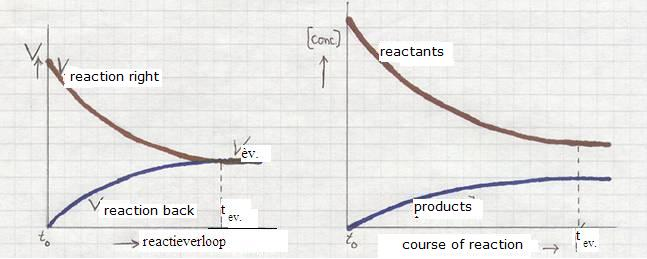How will change the reaction rates of the forward and the backward reaction between start (t0) and finish (the equilibrium is reached (teq)?

The equilibrium condition of a chemical equilibrium is:
The two reaction never become 0 (remain a certain rate) and have equal rates.

Or:      Vforward = Vbackward

Question 2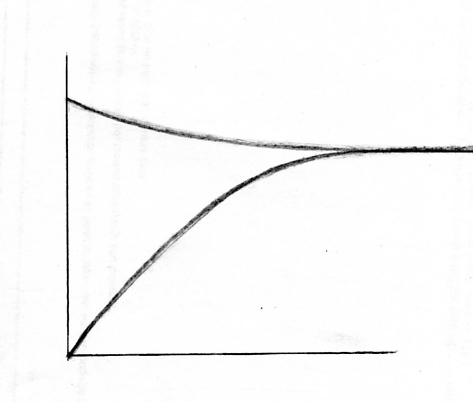1. In a rate diagramme indicate the units at the x-axed and the y-axes.
2. Which substances will dominate duringthe equilibrium, and why so?

### 3.1 A dynamic equilibrium

In fact, every chemical reaction in equilibrium is a dynamic equilibrium
From outside it looks as if nothing happens, but in reality two opposing reactions take place, both with the same rate. They both react; continuously the reactants and the products are busy, but you see nothing.
Such a chemical equilibrium is also called the dynamic equilibrium.

Question 3
A system in chemical equilibrium:
1. has equal amounts of reactants and products
2. does not change the amounts of products and reactants
3. is not influenced by change of temperature
4. can be dislocated by a catalyst

## 4. The equilibrium constant

Vforward = kforward x [I2] x [H2]       and       Vbackward = kbackward x [HI]2

We know that at equilibrium, the forward and the backward reaction occur in the same rate:

Vforward = Vbackward

Or:

kforward x [I2] x [H2] = kbackward x [HI]2Att.: the quotient of the two constants gives one new constant; we may write: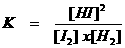K is called: "the equilibrium constant"

Question 4
What will be the value of K (more or less) in the case that the reactants and the products have about the same reaction power?

Question 5
Make a diagramme of the concentrations (see table below) of the reactants and of the products against time.

glucose + fructosesaccharose + water       ΔH < 0

This concerns a homogeneous reaction in equilibrium that started at moment t=0 (in minutes) at constant temperature of 25ºC.

Concentration
of
saccharose
Concentration
of
glucose
Concentration
of
fructose
Duration
of the
reaction
in minutes

1.00
1.00
0
0.20
0.79

60

0.67
120
0.40
0.60

180

240
0.50

300

Draw a graph that shows the change of concentrations of saccharose and fructose versus time

## 5. Le Chatellier & van t Hof

These are the names of two scientists who investigated (1888) the chemical equilibrium and draw some conclusions.
the following rule:

If from outside the system you change one of the conditions of a chemical equilibrium,

this will cause a dislocation of the equilibrium in such a way

that this external change is opposed,
is nihilated as much as possible.

Met andere woorden:

If you disturb a system in equilibrium,

that system will have the tendency to minimalise the effects of that disturbance.

This action continues until a new equilibrium has been reached.

Such an external change, such an influence could be, for example:
• extra amount of one of the substances is added,
• The volume changes,
• or something like that.
1. changing the amount of a substance that participates in an equilibrium
for example: adding Hydrogen ions causes a dislocation of the equilibrium, that will take care that (part of) the extra Hydrogen ions will go away again, by stimulating the forward reaction.
This continues until a new equilibrium is reached.

1.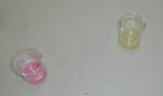In the beaker is NH3(aq) (basic environment) with some drops of the indicator methyl red,
of which the abbreviated formula is: HIn

Than in the solution the following equilibrium will occur:

HInH++ In-
Red                       Yellow

The presence of a base (that absorbs H+) means a dislocation of the equilibrium to the right.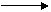the yellow color dominates.

2.Now you add a bit of HCl(aq), the basic environment changes to a less basic or more aced env.

The acid wil donate H+, so:

the equilibrium:   HInH++ In-
Red             Yellow

dislocates to left and the red color will dominate.

3.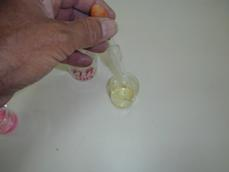Now again we add some base: Nu gaan we weer base toevoegen: (NH3(aq)), the color changes again to yellow

so the same equilibrium dislocated again to the right:

HInH++In-
Red               Yellow

4.Doing this can be repeated endless.
Every time the equilibrium will dislocate to the right of to the left.

The overall situation in the beaker does only change a very littlebit.
There is only a small dislocation of the equilibrium to one of the other side, dependent on the environment.

2. The Volume is changed
A bigger volume means that more space is created for the participating particles in the equilibrium.
The principle is that such a change must be opposed by the equilibrium, and that is possible by creating more particles.
You can change here the equilibrium in different ways:
1. directly in the case of gases, for example by pulling out the piston giving the cylinder more volume.
2. indirectly by diluting in the case of liquids.
In both cases the effect is the same.

example: I2(g) + H2(g)2HI(g) (at elevated temp)
In this example volume change has no influence, because at both sides of the arrows the number of particles is the same. Dislocation is useless.

3. Adding or withdrawing heat energy Adding extra energy to a chemical equilibrium will also result in an attempt to counteract this change. In this case the equilibrium could try to consume that extra added energy, or: stimulate the endothermic reaction.
In the case of HI the equilibrium will dislocate to the left. The forward reaction is exothermic.

[glucose + fructosesaccharose + water ΔH < 0]
The saccharose-equilibrium is exothermic to the right.

At lower temperatures, less energy will be available and the equilibrium will try to 'produce' extra energy.
This can be realised by stimulating the exothermic reaction, the one to the right. Or: at lower temperatures, more saccharose will be formed.
All what was said above is a qualitative view.

Youi can also investigate the same effects in a quantitative way, and prove it with the equilibrium constant K.
That K has a fixed value and does not change, whatever you change at the equilibrium (exept temperature).
Those proves can be delevered with mathematical calculations.Imagine that the amount of I2 is increased.
Look at the mathematical formula for K. If the [I2] increases and K may not change, then, in a mathematical view, the amount of HI must also increase, or: the equilibrium must dislocate.

#### Tollen's Reactant

Ag+-ions are weak oxydators that, for example, can oxydise glucose (the aldehyd group in it).
The equation of that oxydation is: 2Ag++ C6H12O6 + H2O2Ag(s)+ C6H12O7 + 2H+       ΔH > 0       ( eq.1)

To force this equilibrium to the right, you have two options: heating and strengthen the basic environment.

Question 6:
1. Explain those two options.

Basic environment:
Ag+-ions, brought in basic environment, will form a precipitation: AgOH; that soon will form: Ag2O with a brownish color and being a solid.
But: Ag+ reacts only reasonable well as an oxydator when it is free, dissolved; not when it is connected to OH- or to O2-.
If you want to apply this ion as an oxydator, then free ions must be available, even in basic environment.
There is an option to realise that: adding ammonia (NH3(aq)), that can keep the Ag+-ions dissolved in the following way:
Ag+ + 2NH3Ag(NH3)2+ In this way the Ag+-ions are kept in solution, remain dissolved, even in basic environment.

2. Explain how ammonia influences the above equilibrium.
Increase of temperature:
An endothermic reaction costs energy, so: extra energy will support the endothermic reaction.

3. Explain how increase of temperature will influence the equilibrium

Question 7
Some equilibria in the human body - in this case in the blood - are:
1. HHb + O2HbO2 +H+
2. CO2 + H2OH2CO3
3. H2CO3H++ HCO3-
HHb is short for Hemoglobin that in a specific way can donate Hydrogen ions.
What kind of problems can suffer a person with a low pH-value of blood?

Question 8
Ammonia is a gaseous substance that dissolves very well in water:
NH3(g)NH3(aq)
It not only dissolves in water, but it also reacts with water:
NH3 + H2ONH4+ + OH-
The hydroxyde ion is a strong base (much stronger then ammonia), and therefore there is not only a forward, but also a backward reaction.
In the ammonia solution the equilibrium has shifted rather to the left.
1. What will happen after adding:
1. Sodium hydroxyde
2. Hydrochloric acid HCl
2. Explain if the value of K will be large or small
3. Which ones are here the strong and the weaker substances?

Question 9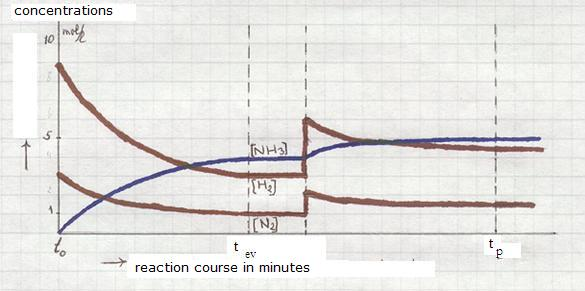1. Analyse the diagramme of the reaction of the gases Hydrogen and Nitrogen, where ammonia(g) was produced (sythesis of Haber-Bosch).
2. What will happen at te moment t0? (at that moment the equilibrium is reached)
3. At the momen tp the equilibrium was disturbed by adding two substances. What substances?

After some time a new equilibrium was reached.
4. What is now the profit in terms of product yield?

### 5.1 Freezing a chemical equilibrium

Suppose you have a system in chemical equilibrium, and you start to cool down (you withdraw heat energy). Normally, and according to the rules of Le Chatellier and van 't Hoff the equilibrium will suffer a shift, a dislocation:
The exothermic reactin (producing energy) will occur extra.

But this is not all there is: adapt to any change always costs some time. Cooling down means for the participating particles that they will move more slowly, and their vibration will also slow down.
Every reaction has its own minimum temperature. Below that temp the reaction does not occur.
But imagine now that you cool down the equilibrium in no timme: within a couple of second the temperature decreases very much (the substances suddenly are thrown in ice, or worse: in liquid Nitrogen).
Then the system has no time to quietly adapt itself to the new situation. The particles immediately lose their movement.
The particles have no longer sufficient energy to participate in the reaction.
If that happens: an reaction in equilibrium is cooled down very quickly, then we say:

the equilibrium was frozen.

The forward and the backward reaction both stop in one moment, and have no time to adapt to the changing circumstances. Products as well as reactants suddenly reach a temperature under their minimum.
After stopping the reactions, also the concentrations do not longer suffer any change. There is no longer a dynamic equilibrium.

Question 10
Explain in you own words why freezing an equilibrium immediately implies the end of the dynamic situation.

Question 11
Bacteria do practically not change when quickly frozen.
All substances remain in not changing concentration and the metabolism remains closed at that cold.
After starting careful heating, many reactions slowly will retake their activity. The bacteria come back to life.

There are people that want to apply this phenomena to themselves: make yourself deeply frozen before dying. Who knows, you could wake up in a hundred years, if new drugs are found for your illness, after being warmed up carefully.
What do you think of that? Technically as well as ethically?

### 5.2 Effects of a catalyst

Question 12
"If an enzym is influencing an equilibrium, the value of the equilibrium constant K will change"

You know that a catalyst can influence the reaction rate of a chemical reaction.
Note: it can be a positive catalyst (increasing the reaction rate), but also a negative one (decreasing the rate).
In the case of a chemical equilibrium, two reaction are being influenced in the same way, with equal effect. The forward reaction is equally in or decreased as the backward reaction.

Question 13
Imagine that the two reactions of an equilibrium are influenced exactly in the same way; Explain if the equilibrium will suffer any shift/dislocation and if the equilibrium remains dynamic.

What is the necessity to apply a catalyst if nothing changes by adding one?
The answer to that question has to do with the time needed to reach an equilibrium. As longas the equilibrium still must be realised (and that may take a long time), such a catalyst can be very usefull, even necessary.
Chemical reactions can be very slow. A catalyst can shorten the period unto te, from hours, days may years, to maybe minutes or seconds.
Some equilibria will never be reached in practice without a catalyst.

In living creatures also are many catalysts: the bio-catalysts or: enzymes.
Most reactions in the metabolism are reactions in equilibrium. So it should be clear that enzymes will be supportive to reach the equilibria in time.
The human body has thousands of different enzymes, all very specific. Without those enzyme, no metabolism, and no life.

Question 14
Design a diagramme that shows the possible influence of a catalyst on the reaction rates within an equilibrium.

## 6. Homogeneous and heterogeneous equilibria

Imagine that all substances participating in an equilibrium are homogeneous substances, than we call that a homogeneous equilibrium. Every substance that participates has any concentration (in the case of solutions and liquids) or pressure (in the case of gases).

The following equilibriuim is heterogeneous: CaCO3(s)CO2(g) + CaO(s)

Imagine a closed reaction room with a certain amount of Calcium carbonate on the bottom. Then this is well heated until the carbonate starts to decompose. The gas Carbon dioxyde and CaO (dry chalk) is produced.
In the reaction cylinder (under the piston) is a chemical equilibrium between the two solids (heterogeneous on the bottom) and the gas (homogeneousin the room). The only substancewith a concentration or pressure is here the gas Carbon dioxyde.
The solids receive in the equation, in the formula for the equilibrium constant the value 1, as said before.

Question 15
Explain the following mathematic expression with the above information: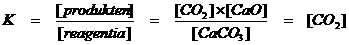Another example of a heterogeneous equilibrium is the dissociation of an insoluble salt in water.
The word 'insoluble' is not enough exact, because there always will be some ions escaping from the lattice when the salt is in the water. The escaped ions (the products) react back to reproduce the salt lattice.

The following equilibrium is realised:
CaCO3(s)Ca2+(aq) +CO32-(aq)

Out of the four components in this mixture, one is solvent and three participate in the proper reaction.

Question 16
Of the three components participating in the chemical reaction, which ones are heterogeneous and which are homogeneous?

Only the ions have a concentration that can be filled out in the equilibrium constant:## 7. Extreme values of K

### 7.1 KC and KP

The equilibrium constant contains the concentrations of the participating homogeneous substances. We add a C: KC.

The other homogeneous situation is in the case of a gas-equilibrium. We do not exactly talk about concentrations, but more about (partial) gas pressure of every participating gas. Every gaseous component in a gas contributes to the pressure.
The partial pressure of a component is proportional with the amount of that gas.
In the formula of the equilibrium constant we fill in the values of those partial pressures; the formule does not really change, but the K is sometimes indicated with KP.

resuming:
• In homogeneous solutions we use KC
• In gases we use KP
• In heterogeneous equilibira of (s)+(aq) we use Ks
• Agreement exist about the 'concentration' of a solid, a heterogeneous substance: it has value 1.
• The value of K does not depend on changes of concentrations, pressure or catalyst.
Changes only depend on temperature change of the equilibrium environment.
There are many equilibria with extreme strong substances at one side an very weak ones at the other side of the arrows.
Again: if equilibrium was reached, de concenctrations at both sides can differ enormously. Almost certainly there will be a lot of the weak and very little of the strong substances.
Such an equilibrium is dislocated, shifted very much to one side.

You can notice that immediately, looking at the formula for K and the value of K: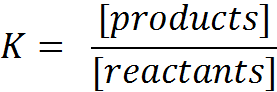Question 17
What is the value of K:
1. In the case of very strong products and very weak reactants?
2. In the case of very weak products and very strong reactants?

### 7.2 KS Solubility product

Salts can dissolve in water well or badly, meaning that many of few free ions are produced, given to the water. The ions of the dissolved salt will reach a certain concentration, and the mathematical product of these ion concentrations we call: the Ions product (Ip).

Question 18
If you dissolve 29 g of NaCl in one liter of water, what is the concentration?

[Na+] = ......... mol/l and [Cl-] = ........ mol/l. Or, the ions product = ............. mol2/l2

About the above mentioned ions and the ions product, they have to do with an equilibrium as soon as badly soluble salts are involved.
Example:
Silver chloride is an insoluble salt (better: badly soluble salt). Totally insoluble salts do not exist. There is always a small amount that dissolves in water
There is always the opportunity for ions in a lattica (at the outer side) to escape and to move into the water.
If they cannot all be set free, because the lattice is too strong, then at the end you reach a situation of equilibrium, where the free ions are in dynamic equilibrium with the lattice. They escape, but as soon as positive and negative ions meet, they join the lattice.

AgCl(s)Ag+(aq)+ Cl-(aq)

Question 19
Mostly the forward reaction in this type of equilibria will be endothermic.
So what happens with the ions product when heating the mixture?

After reaching the equilibrium, and if we keep the temperature constant, the concentrations will no longer change.
(att.: the solid AgCl is heterogeneous and has no concentration (=1)).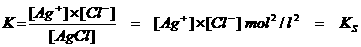Thus every salt with a small solubility will have a KS, called: Solubility product
Imagine you dissolve a tiny littlebit of a badly soluble salt. First there will be an increasing ions product, but soon the maximum value of the Ip will be reached: then the ions product is equal to the solubility product.
Every tablebook has a table with solubility product of salts.
Here we have a table that shows if a salt is more or less soluble.
The salt(s) will reach an equilibrium with its ions(aq) and then the concentrations of the ions will no longer change. The solution has become "saturated": At this temperature no more salt will dissolve.
The ions product has reached its maximum value = the solubility product.

Question 20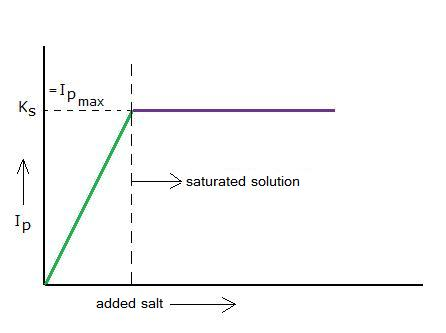Explain the course of the diagramme.

N.B. Do never confuse the solubility product with the actual solubility of a salt!
The solubility is the number of Mols of that salt that will dissolve per liter water (depends on the temperature).

The KS is the product of the ion concentrations of the salt in a saturated solution.

### 7.3 Kw and the autoprotolysis of water

In water solutions always the water molecules are present in incredible amounts and they continuously have contact.
Every watermolecule has a (weak) tendency to donate and to accept protons; this is possible when two water molecules meet:

 H2O + H2OH3O+ + OH- ΔH > 0

Make notice of the fact that both ions are produced in equal amounts. The water equilibrium can only exist in watery environment, and is dislocated very far to one side (the side of the not dissociated molecules), and few ions.
The equilibrium constant will have a low value.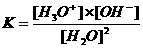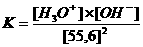Question 21
How is the mathematical relation between K and Kw?

In neutral aquaous solutions at 25ºC, the following will happen:
Of every MOL water molecules (being 6 x 1023) "only" 6 x 1016 have caught a proton and simultaneously, the same amount have donated a proton.

Question 22
How many water molecules participate actively in the proton transfer in 18 grammes of water (=18 ml)?
How many molecules of water are involved?

So, in water of 25ºC the concentration [H3O+] = [OH-] = 10-7 mol/dm3     or:     pH = pOH = 7

Kw (the constant of the autoprotolysis) = K x (55.6)2 = [H3O+] x [OH-] = 10-14 mol2/l2

Question 23
The autoprotolysis of water is an endothermic process. What happens with the equilibrium if water is heated?

Question 24
Affirmation: "Pure boiling water (so at a temp of 100 oC) has no pH=7"
Explain why this theses is true or false.